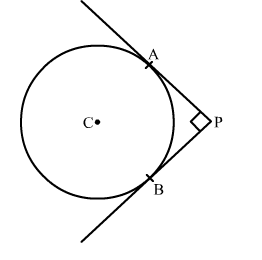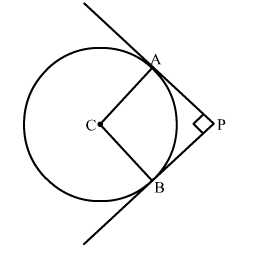# In the given figure, PA and PB are two tangents drawn from an external point P to a circle with centre C and radius 4 cm.`
Question:

In the given figure, PA and PB are two tangents drawn from an external point P to a circle with centre C and radius 4 cm. If  PA ⊥ PB
then the length of each tangent.

(a) 3 cm
(b) 4 cm
(c) 5 cm
(d) 6 cmSolution:

Construction: Join CA and CBWe know that the radius and tangent are perperpendular at their point of contact
∵∠CAP = ∠CBP = 90
Since, in quadrilateral ACBP all the angles are right angles
∴ ACPB is a rectangle
Now, we know that the pair of opposite sides are equal in rectangle
∴ CB = AP and CA = BP
Therefore, CB = AP = 4 cm and CA = BP = 4 cm
Hence, the correct answer is option (b).